# Test: Flow Through Open Channels - 2

## 10 Questions MCQ Test Topicwise Question Bank for Civil Engineering | Test: Flow Through Open Channels - 2

Description
Attempt Test: Flow Through Open Channels - 2 | 10 questions in 30 minutes | Mock test for Civil Engineering (CE) preparation | Free important questions MCQ to study Topicwise Question Bank for Civil Engineering for Civil Engineering (CE) Exam | Download free PDF with solutions
QUESTION: 1

### A right-angled triangular channel symmetrical in section about the vertical carries a discharge of 5 m3/s with a velocity of 1.25 m/s. What is the approximate value of the Froude number of the flow?

Solution:

We know that,
Q = AV
⇒ 5 = A x 1.25 ⇒ A = 4 m2
For a symmetrical right angled triangular channel,
m = 1
∴ A = my2 = 1 x y2 = y2
T = 2my = 2 x 1 x y = 2y
But A = 4 m2
⇒ y2 = 4   ⇒ y = 2 m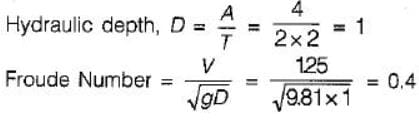QUESTION: 2

### A rectangular channel is 5 m wide and 3 m deep. Water is flowing in the channel for a depth of 2.5 m. The hydraulic radius is

Solution: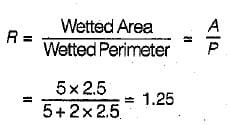QUESTION: 3

### Flow depths across a sluice gate are 2.0 m and 0.5 m. What is the discharge (per metre width)?

Solution: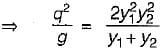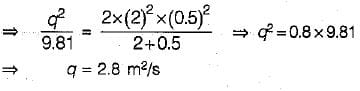QUESTION: 4

Given that
S0 = slope of the channel bottom
Se = slope of the energy line
F = Froude number
the equation of gradually varied flow is expressed as

Solution:
QUESTION: 5

A rectangular channel is 6 m wide and discharges 30 m3s-1. The upstream depth is 2.0 m, acceleration due to gravity is 10 ms-2. Then, what is the specific energy (approximate)?

Solution:

We know that,
Q = AV

⇒ 30 = 6 x 2 x V
⇒  \/ = 2.5 m/s
The specific energy may be given as,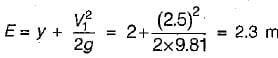QUESTION: 6

Most efficient channel section is

Solution:
QUESTION: 7

For a smooth hump in a subcritical flow to function as a broad crested weir, the height ΔZ of the hump above the bed must satisfy which one of the following?
(E1 = Specific head upstream of the hump, Ec = Specific head at the critical" depth yc) (Neglect friction effects)

Solution:

If the flow is subcritical, then after the introduction of hump the specific energy will decrease by ΔZ (height of hump).
E0 ≤  E1 - ΔZ
⇒ ΔZ ≤ (E1 - E0)

QUESTION: 8

A hydraulic jump occurs at the top of a spillway. The depth before jump is 0.2 m. The sequent depth is 3.2 m. What is the energy dissipated in m (approximate)?

Solution:

The energy dissipation is given by,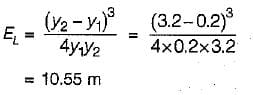QUESTION: 9

What is the normal depth in a wide rectangular channel carrying 0.5 m2/s discharge at a bed slope of 0.0004 and Manning’s n = 0.01?

Solution:

For a wide rectangular channel, the hydraulic radius (F) is approximately equal to the depth of flow. By Manning’s equation, we have,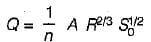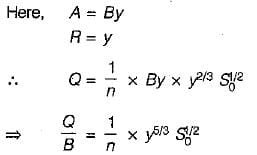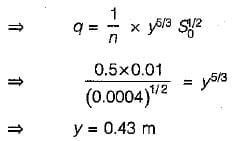QUESTION: 10

Match List-I (Flow section type) with List-ll (Critical discharge is proportional to) where y is the depth of flow-and select the correct answer using the codes given below the lists: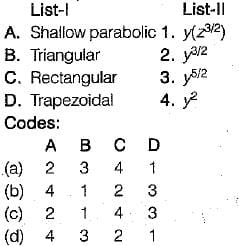Solution:Use Code STAYHOME200 and get INR 200 additional OFF Use Coupon Code